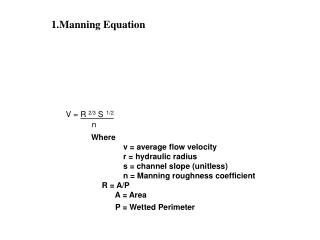Download Presentation1.Manning Equation1.Manning Equation - PowerPoint PPT Presentation

Download Presentation1.Manning Equation
An Image/Link below is provided (as is) to download presentation

Download Policy: Content on the Website is provided to you AS IS for your information and personal use and may not be sold / licensed / shared on other websites without getting consent from its author. While downloading, if for some reason you are not able to download a presentation, the publisher may have deleted the file from their server.

- - - - - - - - - - - - - - - - - - - - - - - - - - - E N D - - - - - - - - - - - - - - - - - - - - - - - - - - -
Presentation Transcript

1. 1.Manning Equation V = R 2/3 S1/2 n Where v = average flow velocity r = hydraulic radius s = channel slope (unitless) n = Manning roughness coefficient R = A/P A = Area P = Wetted Perimeter

2. 1.Manning Equation For the South River: S = 0.0009 n = 0.04 Q = 1.49*A*R 2/3 S1/2 n Where Q = average flow velocity (ft3/s) R = hydraulic radius (ft) S = channel slope (unitless) n = Manning roughness coefficient R = A/P A = Area (ft2) P = Wetted Perimeter (ft)

3. A. Sediment Transport 1. Shear Stress τc = hc ρw g S Shields Equation τc = τ*c (ρs – ρ)gD50 τc = critical boundary shear stress ρs, ρw = grain density (assume 2.65 g/cm3) and water density g = gravitational acceleration (981 cm/s2) D50 = median bed material grain size τ*c = dimensionless critical shear stress (the Shields number) (0.03 for sand; 0.047 for gravel/cobble)

4. A. Sediment Transport 2. Critical Flow Depth τc = hc (ρwgS) hc = critical flow depth to mobilize sediment τc = critical boundary shear stress ρw = water density g = gravitational acceleration (981 cm/s2) S = Average bed slope